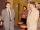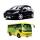Container

First day got off 1/4 water from full container, second day 1/5 of the remaining water. How many percent remain container filled?

Result

p =  60 %

Solution:

1 - 1/4 - 1/5*(1-1/4) = p/100

p = 60

p = 60

Calculated by our simple equation calculator.

Leave us a comment of example and its solution (i.e. if it is still somewhat unclear...):Be the first to comment!To solve this verbal math problem are needed these knowledge from mathematics:

Our percentage calculator will help you quickly calculate various typical tasks with percentages. Do you have a linear equation or system of equations and looking for its solution? Or do you have quadratic equation?

Next similar examples:

1. Tickets1260 tickets sold. On the first day, 80% was sold on the second day was sold. How many tickets were sold first and how much the next day?
2. Two workersTwo workers make 138 parts/items. The first one produces 30% more than the second. How many items will each produce?
3. Two numbersWe have two numbers. Their sum is 140. One-fifth of the first number is equal to half the second number. Determine those unknown numbers.Number 118 divide into two addends, so first addend is 69 greater than 75% of the second addend.
5. Base, percents, valueBase is 344084 which is 100 %. How many percent is 384177?
6. The ballThe ball was discounted by 10 percent and then again by 30 percent. How many percent of the original price is now?Calculate how many percent will increase the length of an HTML document, if any ASCII character unnecessarily encoded as hexadecimal HTML entity composed of six characters (ampersand, grid #, x, two hex digits and the semicolon). Ie. space as: &#x20;
8. PercentsHow many percents is 900 greater than the number 750?
9. Spain vs USASpain lost to the US by 4 goals. In the match total fell 10 goals. How many goals gave the Spain and how the United States?
10. Waiting roomIn the waiting room are people and flies. Together they have 15 heads and 50 legs (fly has 6 legs). How many people and flies are in the waiting room?
11. Parking120 vehicles parking on the morning. Pasenger car is charged 20 CZK, 50 CZK per bus. The guard collected for parking 2640 CZK in total. How many cars and how many buses stood in the parking?
12. Percentage - fractionsAbout what percentage we must increase number 1/6 to get number 1/3?
13. NumberWhat number is 20 % smaller than the number 198?
14. CancerOf the 80 people 50 people ill cancer. What percentage of people isn't ill?
15. Reducing numberReducing the an unknown number by 28.5% we get number 243.1. Determine unknown number.
16. Football match 4In a football match with the Italy lost 3 goals with Germans. Totally fell 5 goals in the match. Determine the number of goals of Italy and Germany.
17. Substitutionsolve equations by substitution: x+y= 11 y=5x-25